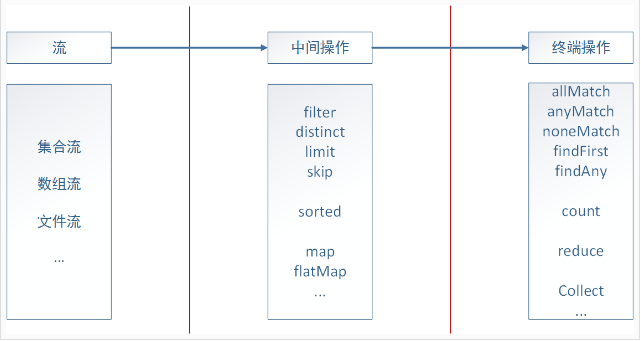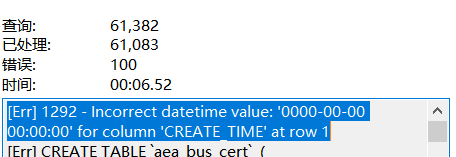Java8 新特性之流式数据处理

## 一. 流式处理简介

``````List<Integer> evens = new ArrayList<>();
for (final Integer num : nums) {
if (num % 2 == 0) {
}
}
``````

``````List<Integer> evens = nums.stream().filter(num -> num % 2 == 0).collect(Collectors.toList());
``````## 二. 中间操作``````public class Student {

/** 学号 */
private long id;

private String name;

private int age;

/** 年级 */

/** 专业 */
private String major;

/** 学校 */
private String school;

// 省略getter和setter
}
````````````// 初始化
List<Student> students = new ArrayList<Student>() {
{
add(new Student(20160001, "孔明", 20, 1, "土木工程", "武汉大学"));
add(new Student(20160002, "伯约", 21, 2, "信息安全", "武汉大学"));
add(new Student(20160003, "玄德", 22, 3, "经济管理", "武汉大学"));
add(new Student(20160004, "云长", 21, 2, "信息安全", "武汉大学"));
add(new Student(20161001, "翼德", 21, 2, "机械与自动化", "华中科技大学"));
add(new Student(20161002, "元直", 23, 4, "土木工程", "华中科技大学"));
add(new Student(20161003, "奉孝", 23, 4, "计算机科学", "华中科技大学"));
add(new Student(20162001, "仲谋", 22, 3, "土木工程", "浙江大学"));
add(new Student(20162002, "鲁肃", 23, 4, "计算机科学", "浙江大学"));
add(new Student(20163001, "丁奉", 24, 5, "土木工程", "南京大学"));
}
};
``````### 2.1 过滤

filter

``````List<Student> whuStudents = students.stream()
.filter(student -> "武汉大学".equals(student.getSchool()))
.collect(Collectors.toList());
``````

distinct
distinct操作类似于我们在写SQL语句时，添加的`DISTINCT`关键字，用于去重处理，distinct基于`Object.equals(Object)`实现，回到最开始的例子，假设我们希望筛选出所有不重复的偶数，那么可以添加distinct操作：

``````List<Integer> evens = nums.stream()
.filter(num -> num % 2 == 0).distinct()
.collect(Collectors.toList());
``````

limit
limit操作也类似于SQL语句中的`LIMIT`关键字，不过相对功能较弱，limit返回包含前n个元素的流，当集合大小小于n时，则返回实际长度，比如下面的例子返回前两个专业为`土木工程`专业的学生：

``````List<Student> civilStudents = students.stream()
.filter(student -> "土木工程".equals(student.getMajor())).limit(2)
.collect(Collectors.toList());
``````

``````List<Student> sortedCivilStudents = students.stream()
.filter(student -> "土木工程".equals(student.getMajor())).sorted((s1, s2) -> s1.getAge() - s2.getAge())
.limit(2)
.collect(Collectors.toList());
``````

skip
skip操作与limit操作相反，如同其字面意思一样，是跳过前n个元素，比如我们希望找出排序在2之后的土木工程专业的学生，那么可以实现为：

``````List<Student> civilStudents = students.stream()
.filter(student -> "土木工程".equals(student.getMajor()))
.skip(2)
.collect(Collectors.toList());
``````

### 2.2 映射

map

``````List<String> names = students.stream()
.filter(student -> "计算机科学".equals(student.getMajor()))
.map(Student::getName).collect(Collectors.toList());
``````

``````int totalAge = students.stream()
.filter(student -> "计算机科学".equals(student.getMajor()))
.mapToInt(Student::getAge).sum();
``````

flatMap
flatMap与map的区别在于 flatMap是将一个流中的每个值都转成一个个流，然后再将这些流扁平化成为一个流 。举例说明，假设我们有一个字符串数组`String[] strs = {"java8", "is", "easy", "to", "use"};`，我们希望输出构成这一数组的所有非重复字符，那么我们可能首先会想到如下实现：

``````List<String[]> distinctStrs = Arrays.stream(strs)
.map(str -> str.split(""))  // 映射成为Stream<String[]>
.distinct()
.collect(Collectors.toList());
``````

``````[j, a, v, a, 8]
[i, s]
[e, a, s, y]
[t, o]
[u, s, e]
``````

distinct只有对于一个包含多个字符的流进行操作才能达到我们的目的，即对`Stream<String>`进行操作。此时flatMap就可以达到我们的目的：

``````List<String> distinctStrs = Arrays.stream(strs)
.map(str -> str.split(""))  // 映射成为Stream<String[]>
.flatMap(Arrays::stream)  // 扁平化为Stream<String>
.distinct()
.collect(Collectors.toList());
``````

flatMap将由map映射得到的`Stream<String[]>`，转换成由各个字符串数组映射成的流`Stream<String>`，再将这些小的流扁平化成为一个由所有字符串构成的大流`Steam<String>`，从而能够达到我们的目的。

## 三. 终端操作

### 3.1 查找

allMatch
allMatch用于检测是否全部都满足指定的参数行为，如果全部满足则返回true，例如我们希望检测是否所有的学生都已满18周岁，那么可以实现为：

``````boolean isAdult = students.stream().allMatch(student -> student.getAge() >= 18);
``````

anyMatch
anyMatch则是检测是否存在一个或多个满足指定的参数行为，如果满足则返回true，例如我们希望检测是否有来自武汉大学的学生，那么可以实现为：

``````boolean hasWhu = students.stream().anyMatch(student -> "武汉大学".equals(student.getSchool()));
``````

noneMathch
noneMatch用于检测是否不存在满足指定行为的元素，如果不存在则返回true，例如我们希望检测是否不存在专业为计算机科学的学生，可以实现如下：

``````boolean noneCs = students.stream().noneMatch(student -> "计算机科学".equals(student.getMajor()));
``````

findFirst
findFirst用于返回满足条件的第一个元素，比如我们希望选出专业为土木工程的排在第一个学生，那么可以实现如下：

``````Optional<Student> optStu = students.stream().filter(student -> "土木工程".equals(student.getMajor())).findFirst();
``````

findFirst不携带参数，具体的查找条件可以通过filter设置，此外我们可以发现findFirst返回的是一个Optional类型，关于该类型的具体讲解可以参考上一篇：Java8新特性 – Optional类

findAny
findAny相对于findFirst的区别在于，findAny不一定返回第一个，而是返回任意一个，比如我们希望返回任意一个专业为土木工程的学生，可以实现如下：

``````Optional<Student> optStu = students.stream().filter(student -> "土木工程".equals(student.getMajor())).findAny();
``````

### 3.2 归约``````// 前面例子中的方法
int totalAge = students.stream()
.filter(student -> "计算机科学".equals(student.getMajor()))
.mapToInt(Student::getAge).sum();
// 归约操作
int totalAge = students.stream()
.filter(student -> "计算机科学".equals(student.getMajor()))
.map(Student::getAge)
.reduce(0, (a, b) -> a + b);

// 进一步简化
int totalAge2 = students.stream()
.filter(student -> "计算机科学".equals(student.getMajor()))
.map(Student::getAge)
.reduce(0, Integer::sum);

// 采用无初始值的重载版本，需要注意返回Optional
Optional<Integer> totalAge = students.stream()
.filter(student -> "计算机科学".equals(student.getMajor()))
.map(Student::getAge)
.reduce(Integer::sum);  // 去掉初始值
``````### 3.3 收集

#### 3.3.1 归约

``````long count = students.stream().collect(Collectors.counting());

// 进一步简化
long count = students.stream().count();
````````````// 求最大年龄
Optional<Student> olderStudent = students.stream().collect(Collectors.maxBy((s1, s2) -> s1.getAge() - s2.getAge()));

// 进一步简化
Optional<Student> olderStudent2 = students.stream().collect(Collectors.maxBy(Comparator.comparing(Student::getAge)));

// 求最小年龄
Optional<Student> olderStudent3 = students.stream().collect(Collectors.minBy(Comparator.comparing(Student::getAge)));
````````````int totalAge4 = students.stream().collect(Collectors.summingInt(Student::getAge));
``````

``````double avgAge = students.stream().collect(Collectors.averagingInt(Student::getAge));
``````

``````IntSummaryStatistics statistics = students.stream().collect(Collectors.summarizingInt(Student::getAge));
``````

``````IntSummaryStatistics{count=10, sum=220, min=20, average=22.000000, max=24}
``````

``````String names = students.stream().map(Student::getName).collect(Collectors.joining());
// 输出：孔明伯约玄德云长翼德元直奉孝仲谋鲁肃丁奉
String names = students.stream().map(Student::getName).collect(Collectors.joining(", "));
// 输出：孔明, 伯约, 玄德, 云长, 翼德, 元直, 奉孝, 仲谋, 鲁肃, 丁奉
``````

#### 3.3.2 分组

``````Map<String, List<Student>> groups = students.stream().collect(Collectors.groupingBy(Student::getSchool));
``````

`groupingBy`接收一个分类器`Function<? super T, ? extends K> classifier`，我们可以自定义分类器来实现需要的分类效果。

``````Map<String, Map<String, List<Student>>> groups2 = students.stream().collect(
Collectors.groupingBy(Student::getSchool,  // 一级分组，按学校
Collectors.groupingBy(Student::getMajor)));  // 二级分组，按专业
``````

``````Map<String, Long> groups = students.stream().collect(Collectors.groupingBy(Student::getSchool, Collectors.counting()));
``````

#### 3.3.3 分区

``````Map<Boolean, List<Student>> partition = students.stream().collect(Collectors.partitioningBy(student -> "武汉大学".equals(student.getSchool())));
````````````public interface Collector<T, A, R> {
/**
* A function that creates and returns a new mutable result container.
*
* @return a function which returns a new, mutable result container
*/
Supplier<A> supplier();

/**
* A function that folds a value into a mutable result container.
*
* @return a function which folds a value into a mutable result container
*/
BiConsumer<A, T> accumulator();

/**
* A function that accepts two partial results and merges them.  The
* combiner function may fold state from one argument into the other and
* return that, or may return a new result container.
*
* @return a function which combines two partial results into a combined
* result
*/
BinaryOperator<A> combiner();

/**
* Perform the final transformation from the intermediate accumulation type
* {@code A} to the final result type {@code R}.
*
* <p>If the characteristic {@code IDENTITY_TRANSFORM} is
* set, this function may be presumed to be an identity transform with an
* unchecked cast from {@code A} to {@code R}.
*
* @return a function which transforms the intermediate result to the final
* result
*/
Function<A, R> finisher();

/**
* Returns a {@code Set} of {@code Collector.Characteristics} indicating
* the characteristics of this Collector.  This set should be immutable.
*
* @return an immutable set of collector characteristics
*/
Set<Characteristics> characteristics();

}
``````## 四. 并行流式数据处理

1）数据大小

2）源数据结构

3）装箱

4）核的数量

5）单元处理开销

1）性能好
ArrayList、数组或IntStream.range，这些数据结构支持随机读取，也就是说它们能轻而易举地被任意分解。
2）性能一般
HashSet、TreeSet，这些数据结构不易公平地被分解，但是大多数时候分解是可能的。
3）性能差blmius
2年前
MySQL:[Err] 1292 - Incorrect datetime value: ‘0000-00-00 00:00:00‘ for column ‘CREATE_TIME‘ at row 1Jacquelyn38
2年前
2020年前端实用代码段，为你的工作保驾护航xxkfz
1年前helloworld_34035044
1年前

​在我们用皕杰报表工具设计填报报表时，如何在新增行里自动增加id呢？能新增整数排序id吗？目前可以在新增行里自动增加id，但只能用uuid函数增加UUID编码，不能新增整数排序id。uuid函数说明：获取一个UUID，可以在填报表中用来创建数据ID语法：uuid()或uuid(sep)参数说明：sep布尔值，生成的uuid中是否包含分隔符''，缺省为Wesley13
1年前
Java日期时间API系列31
时间戳是指格林威治时间1970年01月01日00时00分00秒起至现在的总毫秒数，是所有时间的基础，其他时间可以通过时间戳转换得到。Java中本来已经有相关获取时间戳的方法，Java8后增加新的类Instant等专用于处理时间戳问题。 1获取时间戳的方法和性能对比1.1获取时间戳方法Java8以前Stella981
1年前
Python之time模块的时间戳、时间字符串格式化与转换
Python处理时间和时间戳的内置模块就有time，和datetime两个，本文先说time模块。关于时间戳的几个概念时间戳，根据1970年1月1日00:00:00开始按秒计算的偏移量。时间元组（struct_time），包含9个元素。 time.struct_time(tm_yEaster79
1年前Wesley13
1年前
00：Java简单了解Stella981
1年前Wesley13
1年前
MySQL部分从库上面因为大量的临时表tmp_table造成慢查询Wesley13
Lv1

1.2w

18

1.7k